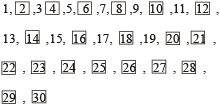## Mathematical Operations Tricks, Examples and Online Test

In such type of questions some relationships are shown with the help of certain symbols/notations and/ or mathematical signs. Each symbol or sign is defined clearly in the question statement itself. In other words, each symbol or sign is accorded two values –one real value and another assigned value of each symbol or sign and then solve the questions accordingly.

For example, Suppose the triangle (∆) means addition.

We know that triangle is a plane figure but here it has been assigned the value of addition (+).

Thus, 3 ∆ 5 ⇒ 3 + 5 = 8

In this way, two work out such questions substitute the assigned/ implied meanings of the symbol or sign and proceed accordingly.

### How to Solve the questions

To solve this type of questions, substitute the real signs in the given expression and then solve the expression according to the BODMAS rule.

Order of Operations – BODMAS

1. 1st. B – Brackets, do all the maths contained in brackets first
2. 2nd. O – Orders, square roots, powers and anything else not listed
3. 3rd. D – Division, do your divisions now
4. 4th. M – Multiplication
5. 5th. A – Addition
6. 6th. S – Subtraction

Example: If + means ÷, × means –, ÷ means × and – means +, then, 8 + 6 × 4 ÷ 3 – 4 = ?

1. – 12
2. – 20/3
3. 12
4. 20/3

Solution. (3): Using the given symbols, we have:

Given expression: = 8 ÷ 6 – 4 × 3 + 4 = 4/3 – 4 × 3 + 4

= 4/3 – 12 + 4 = -20/3.

### Type 1: Value of the Given Expression

Example 1: If ‘÷’ means ‘+’, ‘–’ means ‘÷’, ‘×’ means ‘–’ and ‘+’ means ‘×’ then, 62 ÷ 8 – 4 × 12 + 4 = ?

1. 16
2. 26
3. 1/16
4. 6

Solution: (1) Given expression, 62 ÷ 8 – 4 × 12 + 4 = ?

According to question, after replacement of mathematical sign

62 + 8 ÷ 4 – 12 × 4 = ?

= 64 – 48 = 16

Hence, ? ⇒ 16

### Type 2: Identification of Correct Equation

Example 2: If ‘–’ means ‘+’, ‘+’ means ‘–’, ‘×’ means ‘÷’ and ‘÷’ means ‘×’; then which of the given equations is correct?

1. 30 + 5 – 4 ÷ 10 × 5 = 58
2. 30 + 5 ÷ 4 – 10 × 5 = 22
3. 30 – 5 + 4 ÷ 10 × 5 = 62
4. 30 × 5 – 4 ÷ 10 + 5 = 41

Solution. (4): From option 4:

30 × 5 – 4 ÷ 10 + 5 = 41

According to question, after replacement of mathematical sign

30 ÷ 5 + 4 × 10 – 5 = 41 = 6 + 40 – 5 = 41 = 46 – 5 = 41

Hence option (4) is correct.

## Solved Examples

Example 1: If ‘M’ means ‘÷’, R means ‘+’,  T means ‘-’, and ‘K’ means ‘×’ then what will be the value of the following expression?

20 R 16 K 5 M 10 T8 = ?

1. 36
2. 20
3. 36.5
4. 12

Solution. (2): ? = 20 + 16 × 5 ÷ 10 – 8

or  ? = 20 + 16 ×5/10– 8

or  ? = 20 + 8 – 8 = 20

Example 2: Of the two subjects offered to a class in their final year, 32 students in all are studying Psychology while a total of 26 students are studying Sociology. If 16 students have opted to specialize in both, what is the strength of the class?

1. 74
2. 58
3. 42

Solution. (3): Venn diagram of given information would be as follows.Total strength of the class = 16 + 16 + 10 = 42

Example 3: How many numbers would remain if the numbers which are divisible by 2 and also those having ‘2’ as only one of the digits are dropped from numbers 1 to 30?

1. 14
2. 17
3. 15
4. 10

Solution. (4):